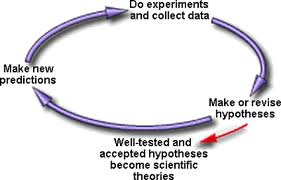# Difference between Hypothesis and Assumption

##### Key Difference: A hypothesis is an uncertain supposition or explanation regarding a phenomenon or event. It is considered to be true by the researcher. An assumption is also a kind of belief which is considered to be true.  A hypothesis must always go through the process of verification and investigation. On the other hand, an assumption may or may not be verified or investigated. In research, assumption denotes the existence of the relationship between the variables. A hypothesis establishes the relationship determined by an assumption.A hypothesis is an uncertain supposition or explanation regarding a phenomenon or event. It is believed to be true by the researcher. It can always be tested by experimentation after the formulation. One acquires all possible evidence to proceed further and the investigation is carried out to test the hypothesis. Initially, it is either accepted or assumed.

According to Tuckman, these three criteria should be kept in mind before stating a hypothesis –

A good hypothesis statement should

• conjecture the direction of the relationship between two or more variables,
• be stated clearly and unambiguously in the form of a declarative sentence, and
• be testable; that is, it should allow restatement in an operational form that can then be evaluated based on dataGenerally, an assumption refers to a belief. An assumption does not require any evidence to support it. It is commonly based on feelings or a hunch. In the following example, the usage of the term 'assumption' is depicted –

'My assumption is that tomorrow Mary will bring snacks for all'.

Assumption and hypothesis often create confusion as both are widely used in the field of research. An assumption is about taking things for granted, without having any firm explanation behind it. On the other hand, hypothesis is a type of assumption for a certain purpose of argument. However, both are not already proved.  An assumption is always assumed to be true. On the other hand, a hypothesis is regarding statements that need certain investigation. In research, assumptions are formulated and on the basis of the assumptions certain hypothesis statements are declared. Thus, a hypothesis can also be considered as an assumption that is taken to be true unless proven otherwise.

Comparison between Hypothesis and Assumption –

 Hypothesis Assumption Definition A Hypothesis is an uncertain explanation regarding a phenomenon or event. It is widely used as a base for conducting tests and the results of the tests determine the acceptance or rejection of the hypothesis. An assumption is also a kind of belief which is considered to be true. An assumption may or may not be verified or investigated. In research, assumption denotes the existence of the relationship between the variables. Origin The term derives from the Greek, hypotithenai meaning "to put under" or "to suppose." from Late Latin assumption-, assumptio taking up, from Latin assumere. Proving methodology Various experiments can lead to various results. Thus a hypothesis can be proved or rejected depending upon the method used by the scientists. General assumptions may or may not require any methods for verification or acceptance. Research assumptions are generally proved by forming hypothesis based on them. Supported by Reasoning Yes Usually Example The higher time the students spend on their studies, the better they achieve tests and score better marks. There is a correlation between the time period to study and marks attained.

Image Courtesy: biology.iupui.edu, b2b-im.com